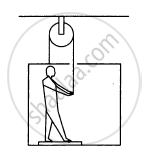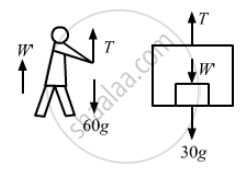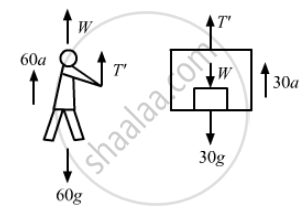# In the Following Figure Shows a Man of Mass 60 Kg Standing on a Light Weighing Machine Kept in a Box of Mass 30 Kg. the Box is Hanging from a Pulley Fixed to the - Physics

Sum

In the following figure shows a man of mass 60 kg standing on a light weighing machine kept in a box of mass 30 kg. The box is hanging from a pulley fixed to the ceiling by a light rope, the other end of which is held by the man himself. If the man manages to keep the box at rest, what is the weight recorded on the machine? What force should he exert on the rope to record his correct weight on the machine?#### Solution

(i) Given, mass of the man = 60 kg
Let W' = apparent weight of the man in this caseFrom the free-body diagram of the man,
W' + T − 60g = 0
⇒ T = 60g − W'    ...(i)

From the free-body diagram of the box,
T − W' = 30g = 0    ...(ii)

From equation (i), we get:
60g − W' − W' − 30g = 0
⇒ W' = 15g
Hence, the weight recorded on the machine is 15 kg.

(ii) To find his actual weight, suppose the force applied by the men on the rope is T because of which the box accelerates upward with an acceleration a'.Here we need to find$\ T'$
Correct weight = W = 60gFrom the free-body diagram of the man,
T' + W − 60g − 60a = 0
⇒ T' − 60a = 0
⇒ T' = 60a    ...(i)

From the free-body diagram of the box,
T' − W − 30g − 30a = 0
⇒ T' −  60g − 30g − 30a = 0
⇒ T' = 30a − 900     ...(ii)

From equations (i) and (ii), we get:
T' = 2T' − 1800
T' = 1800 N
So, the man should exert a force of 1800 N on the rope to record his correct weight on the machine.

Concept: Newton’s Second Law of Motion
Is there an error in this question or solution?

#### APPEARS IN

HC Verma Class 11, Class 12 Concepts of Physics Vol. 1
Chapter 5 Newton's Laws of Motion
Q 39 | Page 82